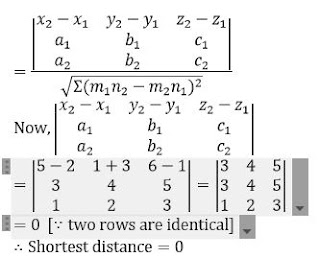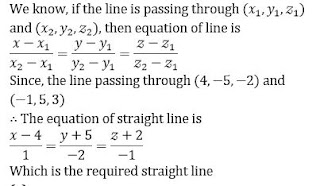##.question { margin-bottom: 20px; border-bottom: 1px solid #ddd; } .ques { font-size: 15px; font-weight: bold; line-height: 150%; } .q-options { padding: 0 !important; list-style: none; margin: 15px 0 !important; } .opt { font-size: 14px; font-weight: normal; } .answer { color: blue; display: none; padding-bottom: 15px; line-height: 150%; } .opt-right.active{ background: #a3ff91; font-size: 19px; line-height: 150%; border: 1px solid ##a3ff91; -webkit-border-radius: 5px; -moz-border-radius: 5px; border-radius: 5px; margin: 10px 0; } .mcq-info { margin: 20px 0 40px; color: #060553; line-height: 150%; } .timer{ overflow: hidden; margin: 20px 0; background: #f7f7f7; padding: 10px; border: 1px solid #eee; border-radius: 3px; } .start-btn, .time-btn, .stop-btn, #reset-start{ border: 1px solid #777; -o-border-radius: 2px; -ms-border-radius: 2px; -webkit-border-radius: 2px; border-radius: 2px; color: #fff; padding: 7px 15px; width: auto; height: auto; line-height: 150%; background: #d12F2F; cursor: pointer; margin-right: 10px; } #reset-start{ background: #0E2230; color: #fff; border-color: #0E2230; display: none; } .start-btn:hover, .time-btn:hover, .stop-btn:hover{ background: #0E2230; color: #fff; border-color: #0E2230; } #reset-start:hover{ background: #d12F2F; color: #fff; border-color: #d12F2F; } @media screen and (max-width: 767px){ .ques, .answer, .mcq-info, .opt{ font-size: 13px; } } Q1. The shortest distance between the lines (x-2)/3=(y+3)/4=(z-1)/5 and (x-5)/1=(y-1)/2=(z-6)/3 is  3  2  1  0 Solution Shortest distance =Q2. The equation of the plane through the points (2, 2, 1) and (9, 3, 6) and perpendicular to the plane 2x+6y+6z-1=0, is  3x+4y+5z=9  3x+4y-5z=9  3x+4y-5z-9=0   None of these Solution B Q3.  The equation of the plane containing the line (x+1)/-3=(y-3)/2=(z+2)/1 and the point 0, 7, -7, is   x+y+z=1  x+y+z=2  None of these  x+y+z=0 Solution The position vector of any point on the given line is i ̂+j ̂+λ(2i ̂+j ̂+4k ̂ )=(2λ+1) i ̂+(λ+1) j ̂+4 λ k ̂ Clearly, this point lies on the plane r ⃗∙(i ̂+2j ̂-k ̂ )=3 Hence, the plane r ⃗∙(i ̂+2j ̂-k ̂ )=3 contains the given line Q4. f the direction ratio of two lines are given by 3lm-4ln+mn=0 and l+2m+3n=0, then the angle between the line is  π/6  π/4  π/3  π/2 Solution Given, 3lm-4ln+mn=0 …..(i) and l+2m+3n=0 …(ii) From Eq. (ii), l=-(2m+3n) putting in Eq. (i) -3(2m+3n)m+4(2m+3n)n+mn=0 ⟹ -〖6m〗^2+〖12n〗^2=0 ⟹m=±√2 n Now,m=√2 n ⟹l=-(2√2 n+3n)=-(2√2+3)n ∴l:m:n=-(3+2√2)n:√2 n:n =-(3+2√2):√2:1 Also,m=-√2 n⟹l=-(-2√2+3)n ∴l:m:n=-(3-2√2)n:-√2:n =-(3-2√2):-√2:1 =cos⁡θ =((3+2√2)(3-2√2)+(√2)(-√2)+1∙1)/(√((3+2√2 )^2+(√2)^2+1^2 ) √((3-2√2)^2+(-√2)^2+1^2 )) =0 ⟹ θ=π/2 Q5.The planex2+y3+z4=1 cuts the coordinate axes in A,B,C then the area of the ∆ABC  √61 sq units  √41 sq units  √29 sq units   None of these Solution  Equation of the plane is x/2+y/3+z/4=1 Here,a=2,b=3,c=4 ∴Area of ∆ABC=1/2 √(a^2 b^2+b^2 c^2+c^2 a^2 ) =1/2 √(2^2∙3^2+3^2∙4^2+4^2∙2^2 ) =1/2 √244=√61 sq units Q6. The equation to the straight line passing through the points (4, -5, -2) and (-1, 5, 3) is  (x+1)/1=(y-5)/2=(z-3)/(-1)  (x-4)/1=(y+5)/(-2)=(z+2)/(-1) x/(-1)=y/5=z/3  x/4=y/(-5)=z/(-2) SolutionQ7.A straight line r=a+λ b meets the plane rn=0 in P. The position vector of P is  a ⃗+[(a ⃗∙n ⃗)/(b ⃗∙n ⃗ )] b ⃗

•  a ⃗-[(a ⃗∙n ⃗)/(b ⃗∙n ⃗ )] b ⃗
•  a ⃗-[(a ⃗∙n ⃗)/(b ⃗∙n ⃗ )] b ⃗
•  None of these
• Solution
The straight line r ⃗=a ⃗+λ b ⃗ meets the plane r ⃗∙n ⃗=0 in P for which λ is given by (a ⃗+λ b ⃗ )∙n ⃗=0⇒λ=-(a ⃗∙n ⃗)/(b ⃗∙n ⃗ ) Thus, the position vector of P is r ⃗=a ⃗((a ⃗∙n ⃗)/(b ⃗∙n ⃗ )) b ⃗ [Putting the value of λ in r ⃗=a ⃗+λb ⃗ ]

Q8.Cosine of the angle between two diagonals of cube is equal to
•  2/√6
•  1/3
•  1/2
•  None of these
Solution
Let OA,OB,OC be the sides of a cube such that OA=OB=OC=a ∴ Direction ratios of OE are (a-0,a-0)ie (a,a,a) ∴Direction cosines of AF are (1/√3,1/√3,1/√3) Similarly, direction of AF are (-1/√3,1/√3,1/√3). ∴ Angle between OE and AF is cos^(-1)⁡〖[-1/√3∙1/√3+1/√3∙1/√3+1/√3∙1/√3] cos^(-1)⁡(1/3) 〗

Q9.The line segment adjoining the points A, B makes projection 1, 4, 3 on x, y, z-axes respectively. Then, the direction cosines of AB are
•  1, 4, 3
•  1/√26,-4/√26,3/√26
•  -1/√26,4/√26,3/√26
•  1/√26,4/√26,3/√26
Solution
DC^' s of AB=1/√(1^2+4^2+3^2 ),4/√(1^2+4^2+3^2 ),3/√(1^2+4^2+3^2 ) =1/√26,4/√26,3/√26

Q10.  A variable plane moves so that sum of the reciprocals of its intercepts on the coordinate axes is 1/2 Then, the plane passes through
•  (1/2,1/2,-1/2)
•  (2, 2, 2)
•  (-1,1,1)
• (0, 0, 0)
Solution
Let x/a+y/b+z/c=1 ….(i) Then,1/a+1/b+1/c=1/2 …(ii) On comparing Eqs. (i) and (ii), we get x=2,y=2,z=2#### Written by: AUTHORNAME

AUTHORDESCRIPTION## Want to know more

Please fill in the details below:

## Latest NEET Articles\$type=three\$c=3\$author=hide\$comment=hide\$rm=hide\$date=hide\$snippet=hide

Name

ltr
item
BEST NEET COACHING CENTER | BEST IIT JEE COACHING INSTITUTE | BEST NEET & IIT JEE COACHING: 3-D COORDINATE GEOMETRY QUIZ-3
3-D COORDINATE GEOMETRY QUIZ-3
https://1.bp.blogspot.com/--9Zn6pBlAhU/X3yN8-gAMiI/AAAAAAAAA54/jjjAcqgDHy8l-1FIxOoZd5u8UGhfik3BQCLcBGAsYHQ/w563-h296/Quiz%2BImage%2BTemplate.jpg
https://1.bp.blogspot.com/--9Zn6pBlAhU/X3yN8-gAMiI/AAAAAAAAA54/jjjAcqgDHy8l-1FIxOoZd5u8UGhfik3BQCLcBGAsYHQ/s72-w563-c-h296/Quiz%2BImage%2BTemplate.jpg
BEST NEET COACHING CENTER | BEST IIT JEE COACHING INSTITUTE | BEST NEET & IIT JEE COACHING
https://www.cleariitmedical.com/2020/10/3-d-coordinate-geometry-quiz-3_6.html
https://www.cleariitmedical.com/
https://www.cleariitmedical.com/
https://www.cleariitmedical.com/2020/10/3-d-coordinate-geometry-quiz-3_6.html
true
7783647550433378923
UTF-8